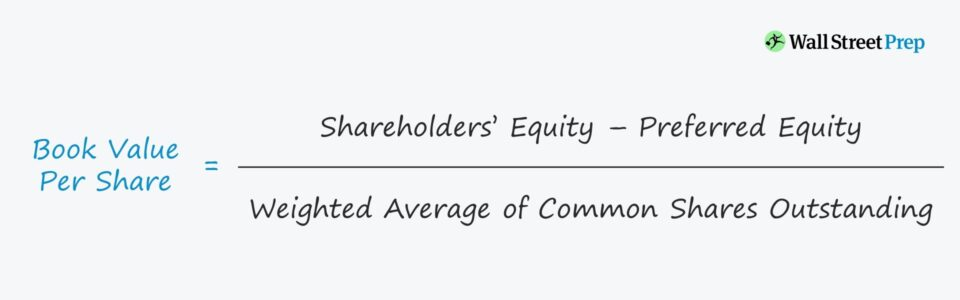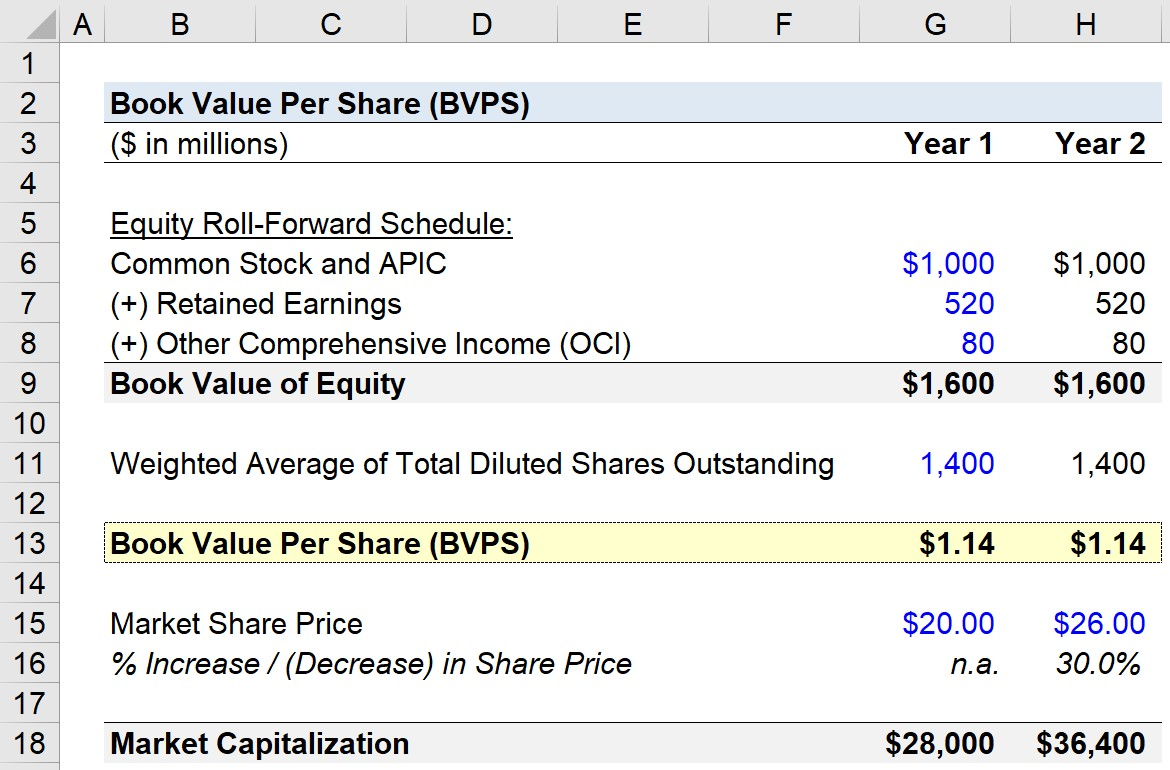Welcome to Wall Street Prep! Use code at checkout for 15% off.# Book Value Per Share (BVPS)

Guide to Understanding Book Value Per Share (BVPS)## How to Calculate Book Value Per Share

The book value per share (BVPS) shows a company’s net asset value (i.e. the total assets minus the total liabilities) on a per-share basis, which makes comparisons among different companies possible.

The book value of equity is defined as the value of a company’s assets as if all of its assets were liquidated to pay off its liabilities. The amount of cash remaining once all outstanding liabilities are paid off is captured by the book value of equity.

Often called shareholder’s equity, the “book value of equity” is an accrual accounting-based profit measure.

As suggested by the name, the “book” value per share calculation begins with finding the necessary balance sheet data from the latest financial report (e.g. 10-K, 10-Q).

## Book Value Per Share Formula

The formula for BVPS involves taking the book value of equity and dividing that figure by the weighted average of shares outstanding.

###### BVPS Formula
• Book Value Per Share = (Shareholders’ Equity – Preferred Equity) / Weighted Average of Common Shares Outstanding

If relevant, the value of preferred equity claims should also be subtracted out from the numerator, the book value of equity.

For example, if a company has a total asset balance of \$40mm and total liabilities of \$25mm, then the book value of equity is \$15mm.

If we assume the company has preferred equity of \$3mm and a weighted average share count of 4mm, the BVPS is \$3.00 (calculated as \$15mm less \$3mm, divided by 4mm shares).

## Book Value Per Share vs. Market Share Price

The difference between book value per share and the market share price is as follows.

• Book Value Per Share (BVPS): The book value of equity per share reflects the equity value recorded on the balance sheet. Unlike the market share price, the BVPS is a historical measure intended for purposes of accrual accounting.
• Market Share Price: The market share price factors in existing investor sentiment regarding future growth and profits (and is forward-looking). In nearly all cases, the market price is much greater than the book value of equity per share. The market share price reflects the most recent prices that investors paid for each share.

Although infrequent, many value investors will see a book value of equity per share below the market share price as a “buy” signal. But an important point to understand is that these investors view this simply as a sign that the company is potentially undervalued, not that the fundamentals of the company are necessarily strong.

In other words, the investors understand the company’s recent performance is underwhelming, but the potential for a long-term turnaround and the rock-bottom price can create a compelling margin of safety.

Nevertheless, most companies with expectations to grow and produce profits in the future will have a book value of equity per share lower than their current publicly traded market share price.

For companies seeking to increase their book value of equity per share (BVPS), profitable reinvestments can lead to more cash.

In return, the accumulation of earnings could be used to reduce liabilities, which results in a higher book value of equity (and BVPS).

Alternatively, another method to increase the BVPS is via share repurchases (i.e. buybacks) from existing shareholders.

## Book Value Per Share Calculator – Excel Model Template

We’ll now move to a modeling exercise, which you can access by filling out the form below.Submitting...

## Book Value Per Share (BVPS) Calculation Example

In our example scenario, the company we’ll be calculating the book value of equity per share with the following financial data:

With those three assumptions given, we can calculate the book value of equity as \$1.6bn.

The next assumption states that the weighted average of common shares outstanding is 1.4bn.

Using those two assumptions, we can calculate the Year 1 BVPS as \$1.14.

• BVPS = \$1.6bn / 1.4bn = \$1.14

As for the next projection period, Year 2, we’ll simply extend each operating assumption from Year 1, and thereby, the BVPS is going to be \$1.14 once again.

The difference lies in the change in the market share price. We’ll assume the trading price in Year 0 was \$20.00, and in Year 2, that the market share price increases to \$26.00, which comes out to be a 30.0% year-over-year increase.

By multiplying the diluted share count of 1.4bn by the corresponding share price for the year, we can calculate the market capitalization for each year.

• Year 1 = \$28.0bn
• Year 2 = \$36.4bn

Despite the increase in share price (and market capitalization), the book value of equity per share remained unchanged.

Unless the company has updated certain assets and liabilities items on its balance sheet to their (usually higher) fair market values (FMVs), the book value of equity will NOT reflect the true picture.

Clear differences between the book value and market value of equity can take place, which occurs more often than not for the vast majority of companies.Step-by-Step Online Course

### Everything You Need To Master Financial Modeling

Enroll in The Premium Package: Learn Financial Statement Modeling, DCF, M&A, LBO and Comps. The same training program used at top investment banks.Inline FeedbacksLearn Financial Modeling Online

Everything you need to master financial and valuation modeling: 3-Statement Modeling, DCF, Comps, M&A and LBO.

X

The Wall Street Prep Quicklesson Series

7 Free Financial Modeling Lessons

Get instant access to video lessons taught by experienced investment bankers. Learn financial statement modeling, DCF, M&A, LBO, Comps and Excel shortcuts.